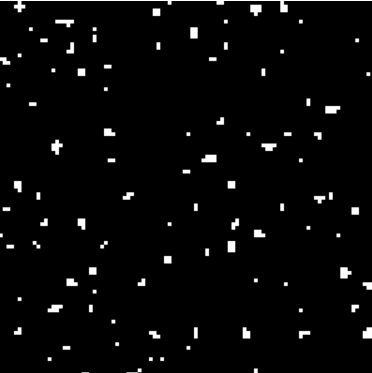# Trees in voxel-based procedurally generated terrain

I am making a minecraft-like game where you build things with block on blocky terrain here. The terrain generates chunk-by-chunk, and I want to add trees to it. Currently, I add trees by using `math.random(100) == 1` on every block during generation, and if it is true then it generates a tree there. But the problem is that it can’t generate trees outside the chunk since it would error. And if I made it so it would just ignore the blocks out of the chunk, I predict that it would cut the tree in half.

For now, I just made it so trees can’t generate on chunk edges. But I want to add a plant frequency slider on the world customization options, and if users turn up the plant frequency high, they will notice that there will never be trees on the chunk edges and it will look weird.

I thought of a solution of using perlin noise to determine if a block is able to generate a tree. If a certain block was above a threshold (e.x. >0.4) then it would mean that the block has a tree on it. Then, if a certain block is near a block with that property, it would determine its Chebyshev distance from the center. If it was 0, then it would generate the trunk, and if it was 1 it would generates the leaves for it. Except it doesn’t work.

In this picture, the white pixels are the “blocks” with a tree on top of it. You can see that the trees would generate in tightly packed clumps.It doesn’t help that I can’t think of any other solutions to this. I would greatly appreicate any help.

Here is my current tree generation code if you want it

``````--plant generation
for x=1, globals.CHUNK_SIZE_X do
for y=1, globals.CHUNK_SIZE_Z do
if x > 1 and x < globals.CHUNK_SIZE_X - 1 and y > 1 and y < globals.CHUNK_SIZE_Z - 1 and math.random(100) == 1 then
local max = math.floor(heightMap[x][y])

if chunk[max][x][y] ~= 0 and max < globals.CHUNK_SIZE_Y - 10 and max > waterLevel + 4 then
--trunk
chunk[max + 1][x][y] = 5
chunk[max + 2][x][y] = 5
chunk[max + 3][x][y] = 5
chunk[max + 4][x][y] = 5
chunk[max + 5][x][y] = 5
--leaves
chunk[max + 6][x][y] = 6
chunk[max + 5][x + 1][y] = 6
chunk[max + 5][x - 1][y] = 6
chunk[max + 5][x][y + 1] = 6
chunk[max + 5][x][y - 1] = 6

chunk[max + 4][x + 1][y] = 6
chunk[max + 4][x - 1][y] = 6
chunk[max + 4][x][y + 1] = 6
chunk[max + 4][x][y - 1] = 6
chunk[max + 4][x + 1][y + 1] = 6
chunk[max + 4][x - 1][y - 1] = 6
chunk[max + 4][x + 1][y - 1] = 6
chunk[max + 4][x - 1][y + 1] = 6
end
end
end
end
``````
1 Like